| Next || Previous || Top |

## Example 15--1D Transport: Kinetic Biodegradation, Cell Growth, and Sorption

A test problem for advective-dispersive-reactive transport was developed by Tebes-Stevens and Valocchi (1997) and Tebes-Stevens and others (1998). Although based on relatively simple speciation chemistry, the solution to the problem demonstrates several interacting chemical processes that are common to many environmental problems: bacterially mediated degradation of an organic substrate; bacterial cell growth and decay; metal sorption; and aqueous speciation, including metal-ligand complexation. In this example, the test problem is solved with PHREEQC, which produces results almost identical to those of Tebes-Stevens and Valocchi (1997) and Tebes-Stevens and others (1998).

The test problem models the transport processes when a pulse of water containing NTA (nitrylotriacetate) and cobalt is injected into a column. The problem includes advection and dispersion in the column, aqueous equilibrium reactions, and kinetic reactions for NTA degradation, growth of biomass, and cobalt sorption.

#### Transport Parameters

The dimensions and hydraulic properties of the column are given in table 38.

Table 38. Hydraulic and physical properties of the column in example 15.

[m, meter; g/m 3 , gram per cubic meter; g/L, gram per liter; m/h, meter per hour]

Property

Value

Length of column

• 10.0 m

Porosity, unitless

• 0.4

Bulk density

• 1.5e6 g/m 3

Grams of sediment per liter (from porosity and bulk density)

• 3.75e3 g/L

Pore-water velocity

• 1.0 m/h

Longitudinal dispersivity

• 0.05 m

#### Aqueous Model

Tebes-Stevens and Valocchi (1997) defined an aqueous model to be used for this test problem that includes the identity of the aqueous species and log K s of the species; activity coefficients were assumed to be 1.0. The database file in table 39 was constructed on the basis of their aqueous model. For the PHREEQC simulation, NTA was defined as a new “element” in the SOLUTION_MASTER_SPECIES data block named “Nta”. From this point on “NTA” will be referred to as “Nta” for consistency with the PHREEQC notation. The gram formula weight of Nta in SOLUTION_MASTER_SPECIES is immaterial if input units are moles in the SOLUTION data block, and is simply set to 1. The aqueous complexes of Nta are defined in the SOLUTION_SPECIES data block. Note that the activity coefficients of all aqueous species are defined with a large value for the a parameter (1×10 7 ) in the -gamma identifier, which forces the activity coefficients to be very nearly 1.0.

 `SOLUTION_MASTER_SPECIES` `C CO2 2.0 61.0173 12.0111` `Cl Cl- 0.0 Cl 35.453` `Co Co+2 0.0 58.93 58.93 ` `E e- 0.0 0.0 0.0` `H H+ -1. 1.008 1.008` `H(0) H2 0.0 1.008` `H(1) H+ -1. 1.008` `N NH4+ 0.0 14.0067 14.0067` `Na Na+ 0.0 Na 22.9898` `Nta Nta-3 3.0 1. 1.` `O H2O 0.0 16.00 16.00` `O(-2) H2O 0.0 18.016` `O(0) O2 0.0 16.00` `SOLUTION_SPECIES` `2H2O = O2 + 4H+ + 4e- ` ` log_k -86.08; -gamma 1e7 0.0` `2 H+ + 2 e- = H2` ` log_k -3.15; -gamma 1e7 0.0` `H+ = H+` ` log_k 0.0; -gamma 1e7 0.0` `e- = e-` ` log_k 0.0; -gamma 1e7 0.0` `H2O = H2O` ` log_k 0.0; -gamma 1e7 0.0` `CO2 = CO2` ` log_k 0.0; -gamma 1e7 0.0` `Na+ = Na+` ` log_k 0.0; -gamma 1e7 0.0` `Cl- = Cl-` ` log_k 0.0; -gamma 1e7 0.0` `Co+2 = Co+2` ` log_k 0.0; -gamma 1e7 0.0` `NH4+ = NH4+` ` log_k 0.0; -gamma 1e7 0.0` `Nta-3 = Nta-3` ` log_k 0.0; -gamma 1e7 0.0` `Nta-3 + 3H+ = H3Nta` ` log_k 14.9; -gamma 1e7 0.0` `Nta-3 + 2H+ = H2Nta-` ` log_k 13.3; -gamma 1e7 0.0` `Nta-3 + H+ = HNta-2` ` log_k 10.3; -gamma 1e7 0.0` `Nta-3 + Co+2 = CoNta-` ` log_k 11.7; -gamma 1e7 0.0` `2 Nta-3 + Co+2 = CoNta2-4` ` log_k 14.5; -gamma 1e7 0.0` `Nta-3 + Co+2 + H2O = CoOHNta-2 + H+` ` log_k 0.5; -gamma 1e7 0.0` `Co+2 + H2O = CoOH+ + H+` ` log_k -9.7; -gamma 1e7 0.0` `Co+2 + 2H2O = Co(OH)2 + 2H+` ` log_k -22.9; -gamma 1e7 0.0` `Co+2 + 3H2O = Co(OH)3- + 3H+` ` log_k -31.5; -gamma 1e7 0.0` `CO2 + H2O = HCO3- + H+` ` log_k -6.35; -gamma 1e7 0.0` `CO2 + H2O = CO3-2 + 2H+` ` log_k -16.68; -gamma 1e7 0.0` `NH4+ = NH3 + H+` ` log_k -9.3; -gamma 1e7 0.0` `H2O = OH- + H+` ` log_k -14.0; -gamma 1e7 0.0` `END `

#### Initial and Boundary Conditions

The background concentrations in the column are listed in table 40. The column contains no Nta or cobalt initially, but has a biomass of 1.36×10 -4 g/L. A flux boundary condition is applied at the inlet of the column, and for the first 20 h (hours), a solution with Nta and cobalt enters the column; the concentrations in the pulse also are given in table 40. After 20 h, the background solution is introduced at the inlet until the experiment ends after 75 h. Na and Cl were not in the original problem definition but were added for charge balancing sorption reactions for PHREEQC (see Sorption Reactions).

#### Kinetic Degradation of Nta and Cell Growth

Nta is assumed to degrade in the presence of biomass and oxygen by the reaction:

```HNta2-
+ 1.62O2
+ 1.272H2
O + 2.424H+
= 0.576C5
H7
O2
N + 3.12H2
CO3
+ 0.424NH4
+
.```
` `

PHREEQC requires kinetic reactants to be defined solely by the moles of each element that enter or leave the solution because of the reaction. Furthermore, the reactants should be charge balanced (no net charge should enter or leave the solution). The Nta reaction converts 1 mol HNta 2- (C 6 H 7 O 6 N) to 0.576 mol C 5 H 7 O 2 N, where the latter is chemically inert, and its concentration can be ignored. The difference in elemental mass contained in these two reactants provides the stoichiometry of the elements C, H, O, and N in the reaction. This stoichiometry is equal to the sum of the elements on the right-hand side of the equation, excluding C 5 H 7 O 2 N, minus the sum of the elements on the left-hand side of the equation. The corresponding change in aqueous element concentrations per mole of HNta 2- reaction is given in table 41 (positive coefficients indicate an increase in aqueous concentration, and negative coefficients indicate a decrease in aqueous concentration).

Table 40. Concentration data for example 15.

[g/L, gram per liter; mol/L, mole per liter; Nta, nitrylotriacetate; ---, absent in pulse]

Constituent

Type

Pulse
concentration

Background
concentration

H +

Aqueous

• 10.0e-6 mol/L
• 10.0e-6 mol/L

Total C

Aqueous

• 4.9e-7 mol/L
• 4.9e-7 mol/L

NH 4 +

Aqueous

• 0.0
• 0.0

O 2

Aqueous

• 3.125e-5 mol/L
• 3.125e-5 mol/L

Nta 3 -

Aqueous

• 5.23e-6 mol/L
• 0.0

Co 2+

Aqueous

• 5.23e-6 mol/L
• 0.0

Na

Aqueous

• 1.0e-3 mol/L
• 1.0e-3 mol/L

Cl

Aqueous

• 1.0e-3 mol/L
• 1.0e-3 mol/L

Biomass

Immobile

• 1.36e-4 g/L

Immobile

• 0.0

Immobile

###### ---
• 0.0

Table 41. Reaction stoichiometry for oxidation of Nta (nitrylotriacetate).

Component

Coefficient

Nta

C

H

O

N

###### 0.424

The following multiplicative Monod rate expression is used to describe the rate of Nta degradation:

###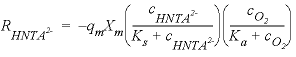, (30)

where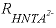is the rate of HNta 2- degradation (mol L -1 h -1 , mole per liter per hour),is the maximum specific rate of substrate utilization (mol/g cells/h),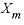is the biomass (g L-1h-1, gram per liter per hour),is the half-saturation constant for the substrate Nta (mol/L),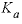is the half-saturation constant for the electron acceptor O 2 (mol/L), and c indicates concentration (mol/L). The rate of biomass production is dependent on the rate of substrate utilization and a first-order decay rate for the biomass:

###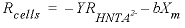, (31)

where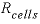is the rate of cell growth (g L-1h-1), Y is the microbial yield coefficient (g cells/mol Nta), and b is the first-order biomass decay coefficient (h -1 ). The parameter values for these equations are listed in table 42.

Table 42. Kinetic rate parameters used in example 15.

[mol, mole; L, liter; g, gram; h, hour]

Parameter

Description

Parameter value

K s

Half-saturation constant for donor

• 7.64e-7 mol/L

K a

Half-saturation constant for acceptor

• 6.25e-6 mol/L

q m

Maximum specific rate of substrate utilization

• 1.418e-3 mol Nta/g cells/h

Y

Microbial yield coefficient

• 65.14 g cells/mol Nta

b

First-order microbial decay coefficient

• 0.00208 h -1

#### Sorption Reactions

Tebes-Stevens and Valocchi (1997) defined kinetic sorption reactions for Co 2+ and CoNta - by the rate equation:

###, (32)

where i is either Co 2+ or CoNta - (mol/L), s i is the sorbed concentration (mol/g sediment),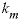is the mass transfer coefficient (h -1 ), and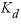is the distribution coefficient (L/g, liter per gram). The values of the coefficients are given in table 43. The values of K d were defined to give retardation coefficients of 20 and 3 for Co 2+ and CoNta - , respectively. Because the sorption reactions are defined to be kinetic, the initial moles of these reactants and the rates of reaction are defined with KINETICS and RATES data blocks; no surface definitions (SURFACE, SURFACE_MASTER_SPECIES, or SURFACE_SPECIES) are needed. Furthermore, all kinetic reactants are immobile, so that the sorbed species are not transported.

Table 43. Sorption coefficients for Co 2+ and CoNta - .

[h, hour; L/g, liter per gram]

Species

k m

K d

Co 2+

CoNta -

###### 5.33e-4 L/g

When modeling with PHREEQC, kinetic reactants must be charge balanced. For sorption of Co 2+ and CoNta - , 1 mmol of NaCl was added to the solution definitions to have counter ions for the sorption process. The kinetic sorption reactions were then defined to remove or introduce (depending on the sign of the mole transfer) CoCl 2 and NaCoNta, which are charge balanced. To convert from moles sorbed per gram of sediment ( s i ) to moles sorbed per liter of water, it is necessary to multiply by the grams of sediment per liter of water, 3.75×10 3 g/L.

#### Input File

See Input file for example 15. shows the input file derived from the preceding problem definition. Although rates have been given in units of mol L-1h-1, rates in PHREEQC are always mol/s (mole per second), and all rates have been adjusted to seconds in the definition of rate expressions in the input file. The density of water is assumed to be 1 kg/L.

 `DATABASE ex15.dat` `TITLE Example 15.--1D Transport: Kinetic Biodegradation, Cell Growth, and Sorption` `***********` `PLEASE NOTE: This problem requires database file ex15.dat!!` `***********` `PRINT` ` -reset false` ` -echo_input true` ` -status false` `SOLUTION 0 Pulse solution with NTA and cobalt` ` units umol/L` ` pH 6` ` C .49` ` O(0) 62.5 ` ` Nta 5.23` ` Co 5.23` ` Na 1000` ` Cl 1000` `SOLUTION 1-10 Background solution initially filling column` ` units umol/L` ` pH 6` ` C .49` ` O(0) 62.5 ` ` Na 1000` ` Cl 1000` `COPY solution 0 100 # for use later on, and in` `COPY solution 1 101 # 20 cells model` `END` `RATES Rate expressions for the four kinetic reactions` `#` ` HNTA-2` ` -start` `10 Ks = 7.64e-7` `20 Ka = 6.25e-6` `30 qm = 1.407e-3/3600` `40 f1 = MOL("HNta-2")/(Ks + MOL("HNta-2"))` `50 f2 = MOL("O2")/(Ka + MOL("O2"))` `60 rate = -qm * KIN("Biomass") * f1 * f2 ` `70 moles = rate * TIME` `80 PUT(rate, 1) # save the rate for use in Biomass rate calculation` `90 SAVE moles` ` -end` `#` ` Biomass` ` -start` `10 Y = 65.14` `20 b = 0.00208/3600` `30 rate = GET(1) # uses rate calculated in HTNA-2 rate calculation` `40 rate = -Y*rate -b*M` `50 moles = -rate * TIME` `60 if (M + moles) < 0 then moles = -M` `70 SAVE moles` ` -end` `#` ` Co_sorption` ` -start` `10 km = 1/3600` `20 kd = 5.07e-3` `30 solids = 3.75e3` `40 rate = -km*(MOL("Co+2") - (M/solids)/kd)` `50 moles = rate * TIME` `60 if (M - moles) < 0 then moles = M` `70 SAVE moles` ` -end` `#` ` CoNta_sorption` ` -start` `10 km = 1/3600` `20 kd = 5.33e-4` `30 solids = 3.75e3` `40 rate = -km*(MOL("CoNta-") - (M/solids)/kd)` `50 moles = rate * TIME` `60 if (M - moles) < 0 then moles = M` `70 SAVE moles` ` -end` `KINETICS 1-10 Four kinetic reactions for all cells` ` HNTA-2` ` -formula C -3.12 H -1.968 O -4.848 N -0.424 Nta 1.` ` Biomass` ` -formula H 0.0` ` -m 1.36e-4` ` Co_sorption` ` -formula CoCl2` ` -m 0.0` ` -tol 1e-11` ` CoNta_sorption` ` -formula NaCoNta` ` -m 0.0` ` -tol 1e-11` `COPY kinetics 1 101 # to use with 20 cells ` `END` `SELECTED_OUTPUT` ` -file ex15.sel` ` -mol Nta-3 CoNta- HNta-2 Co+2` `USER_PUNCH` ` -headings hours Co_sorb CoNta_sorb Biomass` ` -start` ` 10 punch TOTAL_TIME/3600 + 3600/2/3600` ` 20 punch KIN("Co_sorption")/3.75e3` ` 30 punch KIN("CoNta_sorption")/3.75e3` ` 40 punch KIN("Biomass")` `USER_GRAPH 1 Example 15` ` -headings 10_cells: Co+2 CoNTA- HNTA-2 pH` ` -chart_title "Kinetic Biodegradation, Cell Growth, and Sorption: Dissolved Species"` ` -axis_titles "Time, in hours" "Micromoles per kilogram water" "pH"` ` -axis_scale x_axis 0 75` ` -axis_scale y_axis 0 4` ` -axis_scale secondary_y_axis 5.799 6.8 0.2 0.1` ` -plot_concentration_vs t` ` -start` ` 10 x = TOTAL_TIME/3600 + 3600/2/3600` ` 20 PLOT_XY -1, -1, line_width = 0, symbol_size = 0` ` 30 PLOT_XY x, MOL("Co+2") * 1e6, color = Red, line_width = 0, symbol_size = 4` ` 40 PLOT_XY x, MOL("CoNta-") * 1e6, color = Green, line_width = 0, symbol_size = 4` ` 50 PLOT_XY x, MOL("HNta-2") * 1e6, color = Blue, line_width = 0, symbol_size = 4` ` 60 PLOT_XY x, -LA("H+"), y-axis = 2, color = Magenta, line_width = 0, symbol_size = 4` ` -end` `USER_GRAPH 2 Example 15` ` -headings 10_cells: Co+2 CoNTA- Biomass` ` -chart_title "Kinetic Biodegradation, Cell Growth, and Sorption: Sorbed Species"` ` -axis_titles "Time, in hours" "Nanomoles per kilogram water" \` ` "Biomass, in milligrams per liter"` ` -axis_scale x_axis 0 75` ` -axis_scale y_axis 0 2` ` -axis_scale secondary_y_axis 0 0.4` ` -plot_concentration_vs t` ` -start` ` 10 x = TOTAL_TIME/3600 + 3600/2/3600` ` 20 PLOT_XY -1, -1, line_width = 0, symbol_size = 0` ` 30 PLOT_XY x, KIN("Co_sorption") / 3.75e3 * 1e9, color = Red, line_width = 0, symbol_size = 4` ` 40 PLOT_XY x, KIN("CoNta_sorption") / 3.75e3 * 1e9, color = Green, line_width = 0, \` ` symbol_size = 4` ` 50 PLOT_XY x, KIN("Biomass") * 1e3, y-axis = 2, color = Magenta, line_width = 0, \` ` symbol_size = 4` ` -end -end` `TRANSPORT First 20 hours have NTA and cobalt in infilling solution` ` -cells 10` ` -lengths 1` ` -shifts 20` ` -time_step 3600` ` -flow_direction forward` ` -boundary_conditions flux flux` ` -dispersivities .05` ` -correct_disp true` ` -diffusion_coefficient 0.0` ` -punch_cells 10` ` -punch_frequency 1` ` -print_cells 10` ` -print_frequency 5` ` ` `COPY solution 101 0 # initial column solution becomes influent` `END` `TRANSPORT Last 55 hours with background infilling solution` ` -shifts 55` `COPY cell 100 0 # for the 20 cell model...` `COPY cell 101 1-20` `END` `USER_PUNCH` ` -start` ` 10 punch TOTAL_TIME/3600 + 3600/4/3600` ` 20 punch KIN("Co_sorption")/3.75e3` ` 30 punch KIN("CoNta_sorption")/3.75e3` ` 40 punch KIN("Biomass")` ` -end` `USER_GRAPH 1` ` -headings 20_cells: Co+2 CoNTA- HNTA-2 pH` ` -start` ` 10 x = TOTAL_TIME/3600 + 3600/4/3600` ` 20 PLOT_XY -1, -1, line_width = 0, symbol_size = 0` ` 30 PLOT_XY x, MOL("Co+2") * 1e6, color = Red, symbol_size = 0` ` 40 PLOT_XY x, MOL("CoNta-") * 1e6, color = Green, symbol_size = 0` ` 50 PLOT_XY x, MOL("HNta-2") * 1e6, color = Blue, symbol_size = 0` ` 60 PLOT_XY x, -LA("H+"), y-axis = 2, color = Magenta, symbol_size = 0` ` -end` `USER_GRAPH 2` ` -headings 20_cells: Co+2 CoNTA- Biomass` ` -start` ` 10 x = TOTAL_TIME/3600 + 3600/4/3600` ` 20 PLOT_XY -1, -1, line_width = 0, symbol_size = 0` ` 30 PLOT_XY x, KIN("Co_sorption") / 3.75e3 * 1e9, color = Red, symbol_size = 0` ` 40 PLOT_XY x, KIN("CoNta_sorption") / 3.75e3 * 1e9, color = Green, symbol_size = 0` ` 60 PLOT_XY x, KIN("Biomass") * 1e3, y-axis = 2, color = Magenta, symbol_size = 0` ` -end ` `TRANSPORT First 20 hours have NTA and cobalt in infilling solution` ` -cells 20` ` -lengths 0.5` ` -shifts 40` ` -initial_time 0` ` -time_step 1800` ` -flow_direction forward` ` -boundary_conditions flux flux` ` -dispersivities .05` ` -correct_disp true` ` -diffusion_coefficient 0.0` ` -punch_cells 20` ` -punch_frequency 2` ` -print_cells 20` ` -print_frequency 10` `COPY cell 101 0` `END` `TRANSPORT Last 55 hours with background infilling solution` ` -shifts 110` `END`

The 10-meter column was discretized with 10 cells of 1 meter each. The first two SOLUTION data blocks (table 44) define the infilling solution and the initial solution in cells 1 through 10. The solutions are copied to solution 100 and 101, to be used later in the 20-cell model.

The RATES data block defines the rate expressions for four kinetic reactions: HNta-2, Biomass, Co_sorption, and CoNta_sorption. The rate expressions are initiated with -start , defined with numbered Basic-language statements, and terminated with -end . The last statement of each expression is SAVE followed by a variable name. This variable is the number of moles of reaction over the time subinterval and is calculated from an instantaneous rate (mol/s) times the length of the time subinterval (s), which is given by the variable “TIME”. Lines 30 and 20 in the first and second rate expressions and line 10 in the third and fourth rate expressions adjust parameters to units of seconds from units of hours. The function “MOL” returns the concentration of a species (mol/kgw), the function “M” returns the moles of the reactant for which the rate expression is being calculated, and “KIN” returns the moles of the specified kinetic reactant. The functions “PUT” and “GET” are used to save and retrieve a term that is common to both the HNta-2 and Biomass rate expressions (see also See Reaction-Path Calculations).

The KINETICS data block defines the names of the rate expressions that apply to each cell; cells 1 through 10 are defined simultaneously in this example. For each rate expression that applies to a cell, the formula of the reactant ( -formula ) and the moles of the reactant initially present ( -m , if needed to be different from the default of 1 mol) are defined. It is also possible to define a tolerance ( -tol ), in moles, for the accuracy of the numerical integration for a rate expression. Note that the HNta-2 rate expression generates a negative rate, so that elements with positive coefficients in the formula are removed from solution and negative coefficients add elements to solution. The biomass reaction adds “H 0.0”, or zero moles of hydrogen; in other words, it does not add or remove anything from solution. The assimilation of carbon and nutrients that is associated with biomass growth is ignored in this simulation. Also, the kinetics block is copied to KINETICS 101, for use in the 20-cell model.

The SELECTED_OUTPUT data block punches the molalities of the aqueous species Nta-3, CoNta-, HNta-2 and Co+2 to the file ex15.sel . To each line in the file, the USER_PUNCH data block appends the time (in hours), the sorbed concentrations converted to mol/g sediment, and the biomass. USER_GRAPH plots the concentrations as symbols without lines to facilitate the comparison with the 20-cell model.

The first TRANSPORT data block defines the first 20 h of the experiment, during which Nta and cobalt are added at the column inlet. The column is defined to have 10 cells ( -cells ) of length 1 m ( -lengths ). The duration of the advective-dispersive transport simulation is 20 time steps ( -shifts ) of 3,600 seconds ( -time_step ). The direction of flow is forward ( -flow_direction ). Each end of the column is defined to have a flux boundary condition ( -boundary_conditions ). The dispersivity is 0.05 m ( -dispersivities ) and the diffusion coefficient is set to zero ( -diffusion_coefficient ). Data are written to the selected-output file only for cell 10 ( -punch_cells ) after each shift ( -punch_frequency ), and data are written to the output file only for cell 10 ( -print_cells ) after each fifth shift ( -print_frequency ).

At the end of the first advective-dispersive transport simulation, the initial column solution, which was stored as SOLUTION 101, is copied to SOLUTION 0, to become the influent for the next transport simulation. The second TRANSPORT data block defines the final 55 h of the experiment, during which Nta and cobalt are not present in the infilling solution. All parameters are the same as in the previous TRANSPORT data block; only the number of transport steps ( -shifts ) is increased to 55.

#### Grid Convergence

With advective-dispersive-reactive transport simulations, it is always necessary to check the numerical accuracy of the results. In general, analytical solutions will not be available for these complex simulations, so the only test of numerical accuracy is to refine the grid and time step, rerun the simulation, and compare the results. If simulations on two different grids give similar results, there is some assurance that the numerical errors are relatively small. If simulations on two different grids give significantly different results, the grid must be refined again and the process repeated. Unfortunately, doubling the grid size at least quadruples the number of solution calculations that must be made because the number of cells doubles and the time step is halved. If the cell size approaches the size of the dispersivity, it may require even more solution calculations because the number of mix steps in the dispersion calculation will increase as well.

To test grid convergence in this example, the number of cells in the column were doubled. All keyword data blocks that defined compositions for the range 1 through 10 were changed to 1 through 20. In addition, the parameters for advective-dispersive transport were adjusted to be consistent with the new number of cells. The final TRANSPORT data block in table 44 defines the 20-cell model. The number of cells and number of shifts are doubled; the cell length and time step are halved. To print information for the same location as the 10-cell model (the end of the column), the -punch_cells and -print_cells are set to cell 20. To print information at the same time in the simulation as the 10-cell model, -punch_frequency is set to every 2 shifts, -print_frequency is set to every 10 shifts, and the time step for going from the cell-midpoint to the column-end is halved on line 10 in USER_PUNCH.

#### Results

The distributions of aqueous and immobile constituents in the column at the end of 75 h are shown in figures See Dissolved concentrations and pH values at the outlet of the column for Nta and cobalt transport simulations with 10 (symbols) and 20 cells (lines). and See Concentrations of sorbed species and biomass at the outlet of the column for Nta and cobalt transport simulations with 10 (symbols) and 20 cells (lines). for the 10- and 20-cell models. In the experiment, two pore volumes of water with Nta and cobalt were introduced to the column over the first 20 h and then followed by 5.5 pore volumes of background water over the next 55 h. At 10 h, HNta 2- begins to appear at the column outlet along with a rise in the pH (fig. 16). If Nta and cobalt were conservative and dispersion were negligible, the graph would show square pulses that increase at 10 h and decrease at 30 h. However, the movement of the Nta and cobalt is retarded relative to conservative movement by the sorption reactions, and small concentrations arrive early because of dispersion. The peak in Nta and cobalt concentrations occurs in the CoNta - complex between 30 and 40 h. The peak in Co 2+ concentration is even more retarded by its sorption reaction and does not show up until near the end of the experiment.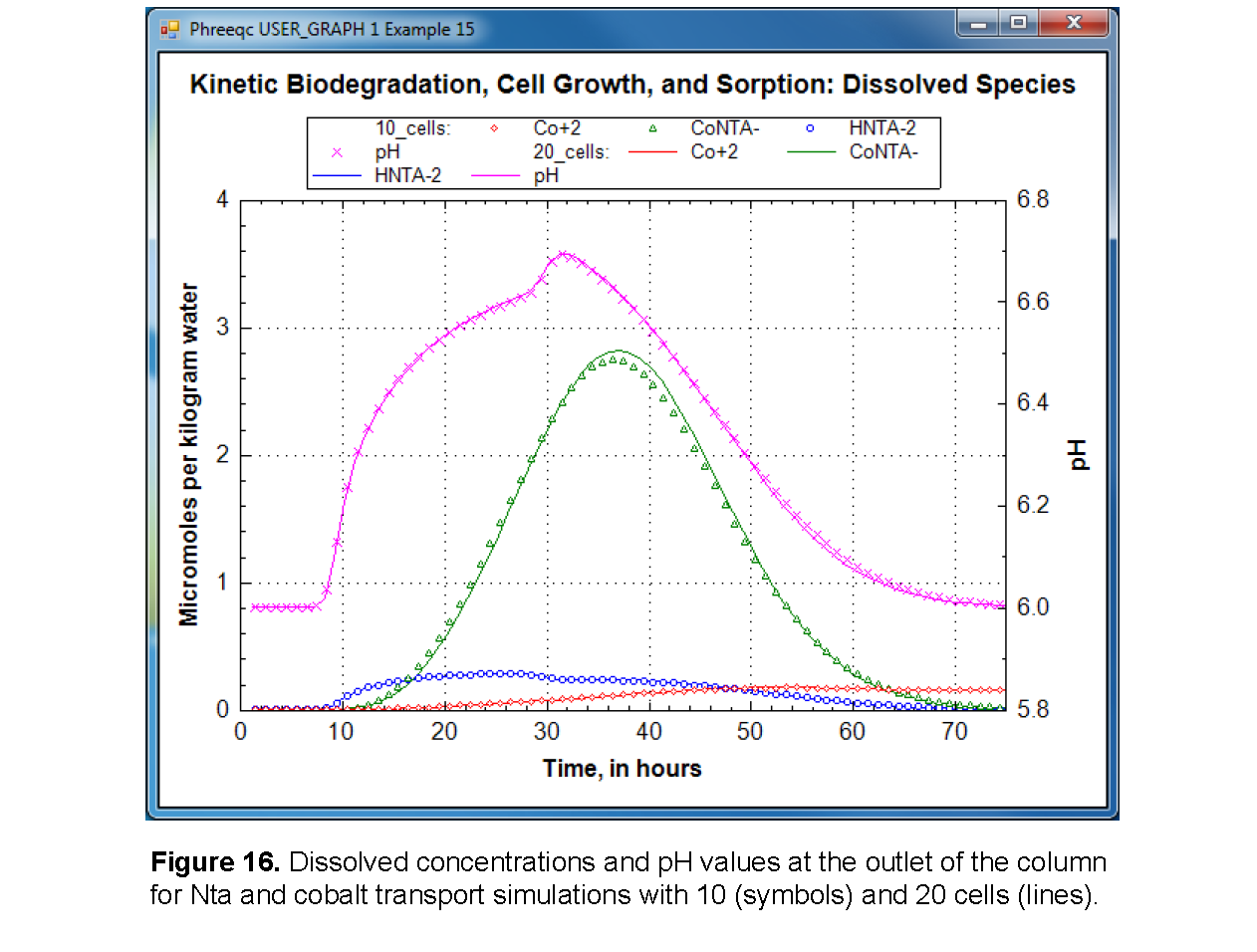In figure 17, solid-phase concentrations are plotted against time for concentrations in the last cell of the column. The sorbed CoNta - concentration peaks between 30 and 40 h and lags slightly behind the peak in the dissolved concentration of the CoNta - complex. Initially, no Nta is present in the column and the biomass decreases slightly over the first 10 h because of the first-order decay rate for the biomass. When the Nta moves through the cells, the biomass increases because the Nta becomes available as substrate for microbes. After the peak in Nta has moved through the column, biomass concentrations level off and then begin to decrease because of decay. The K d for cobalt sorption gives a greater retardation coefficient than the K d for CoNta - sorption, and the sorbed concentration of Co 2+ appears to be still increasing at the end of the experiment.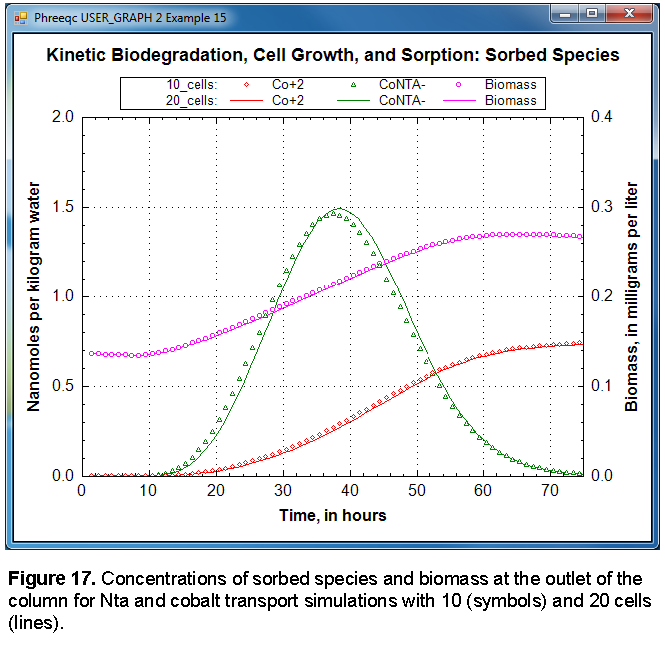Both the 10-cell and the 20-cell models give similar results, which indicates that the numerical errors in the advective-dispersive transport simulation are relatively small; furthermore, the results are very similar to results given by Tebes-Stevens and Valocchi (1997) and Tebes-Stevens and others (1998). However, Tebes-Stevens and Valocchi (1997) included another part to their test problem that increased the rate constants for the sorption reactions from 1 to 1,000 h -1 . The increased rate constants generate a stiff set of partial differential equations, which are equations that describe processes that occur on very different time scales. The stiff problem, with very fast sorption reactions, proved intractable for the explicit Runge-Kutta algorithm, but can be solved with the -cvode algorithm. With -cvode, the calculation of the slow sorption column takes about four times longer than with Runge-Kutta and calculation of the fast sorption column is about six times longer than for slow sorption. Another way to solve the stiff problem is to introduce the fast kinetic sorption reaction as an equilibrium process, which constitutes instantaneous rates. However, even with equilibrium sorption, grid convergence was computationally much more intensive; it was necessary to use 100 cells or more to arrive at a satisfactory solution. As an estimate of relative CPU times, the 20-cell model took 2.7 times the CPU time of the 10-cell model. A 200-cell model took approximately 600 times the CPU time of the 10-cell model.

| Next || Previous || Top |